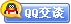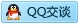##用户名 Email 自动登录 找回密码 密码 会员注册
 VIP会员，3年作业免费下 ！ 奥鹏作业，奥鹏毕业论文检测 新手作业下载教程，充值问题 没有找到答案，请在此处留言！ 2019年09月最新全国统考资料 投诉建议，加盟合作！奥鹏课程积分软件(ver:3.1)

# 东大19秋学期《高级语言程序设计基础》在线平时作业2（参考）发表于 2019-9-7 04:11:09 | 显示全部楼层 |阅读模式【奥鹏】[东北大学]19秋学期《高级语言程序设计基础》在线平时作业2 试卷总分:100    得分:100 第1题,当正常执行了文件关闭操作时，fclose函数的返回值是（ ）。 A、-1 B、随机值 C、0 D、1 正确答案: 第2题,判断字符串a和b是否相等，应当使用（ ）。 A、if(a==b) B、if(a=b) C、if(strcpy(a,b)) D、if(strcmp(a,b))" 正确答案: 第3题,以下能正确定义整型变量x、y和z并为其赋初值5的语句是（ ）。 A、int x=y=z=5; B、int x,y,z=5; C、int x=5,y=5,z=5; D、x=5,y=5,z=5;" 正确答案: 第4题,有字符数组a和b，则正确的输出语句是（ ）。 A、puts(a.b); B、printf("%s,%s",a[],b[]); C、pitchar(a,b); D、puts(a); puts(b); 正确答案: 第5题,下面程序的运行结果是（ ）。 char a="abcdef"; char b="ABC"; strcpy(a,b); printf("%c",a); A、输出空格 B、\0 C、e D、f 正确答案: 第6题,以下程序执行后a的值为（ ）。main( ){ int a，m=2, k=1, *p=&k;a=p!=&m;printf("%d\n",a);　　} A、-1 B、0 C、1 D、2 正确答案: 第7题,设a、b、c、d均是int类型变量，为了使以下程序段的输出为：1234+123+12+1，正确的输入形式应当是（ ）。 scanf("%4d+%3d+%2d+%1d",&a,&b,&c,&d);printf("%4d+%3d+%2d+%1d",a, b, c, d); A、1234123121 B、1234123412341234 C、1234+1234+1234+1234 D、1234+123+12+1 正确答案: 第8题,在C语言中，错误的int类型的常数是（ ）。 A、32768 B、0 C、37 D、0xAF 正确答案: 第9题,以下对指针变量的操作中，不正确的是（ ）。 A、int p, *q; q=&p; B、int *p, *q; q=p=NULL; C、int a=5, *p; *p=a; D、int a=5, *p=&a, *q=&a; *p=*q;" 正确答案: 第10题,以下能正确读入字符串的程序段是（ ）。 A、char *p; scanf("%s", p); B、char str; scanf("%s", &str); C、char str, *p; p=str; scanf("%s", p); D、char str, *p=str; scanf("%s", p);" 正确答案: 第11题,以下运算正确的程序段是（ ）。 A、char str1[]="ABCDE", str2[]="abcdef"; strcpy(str1, str2); B、char str, *st="abcde"; strcat(str, st); C、char *st1="ABCDS", *st2="abcde"; strcat(st1,st2); D、char str="", *st="abcde"; strcat(str, st);" 正确答案: 第12题,以下正确的说法是（ ）。 A、实参和与其对应的形参各占用独立的存储单元 B、实参和与其对应的形参共占用一个存储单元 C、只有当实参和与其对应的形参同名时才共用存储单元 D、形参是虚拟的，不占用存储单元 正确答案: 第13题,语句while (!y)中的表达式 !y 等价于（ ）。 A、y==0 B、y!=1 C、y!=0 D、y==1 正确答案: 第14题,在一个源文件中定义的全局变量的作用域为（ ）。 A、本文件的全部范围 B、本程序的全部范围 C、本函数的全部范围 D、从定义该变量的位置开始至本文件结束 正确答案: 第15题,若有以下说明，并且0≤i10,则（ ）是对数组元素的错误引用。int a[]={1, 2, 3, 4, 5, 6, 7, 8, 9, 10}, *p=a, I; A、*(a+i) B、a[p-a+i] C、p+i D、*(&a[i]) 正确答案: 第16题,C语言规定：简单变量做实参时，它和对应形参之间的数据传递方式是（ ）。 A、地址传递 B、单向值传递 C、由实参传给形参，再由形参传回实参 D、由用户指定传递方式 正确答案: 第17题,以下程序的输出结果是（ ）。 main() { int a=0,b=1,c=0,d=20； if(a) d=d-10； else if(!b) if(!c) d=15； else d=25； printf("d=%d\n",d)；} A、d=10 B、d=15 C、d=20 D、d=25 正确答案: 第18题,已知intx=10,y=20,z=30;以下语句执行后x,y,z的值是（ ）。if(xy)z=x;x=y;y=z; A、x=10,y=20,z=30 B、x=20,y=30,z=30 C、x=20,y=30,z=10 D、x=20,y=30,z=20" 正确答案: 第19题,以下正确的函数说明是（ ）。 A、int fun(int a, b) B、float fun(int a; int b) C、double fun( ) D、int fun(char a[ ][ ]) 正确答案: 第20题,设ch是char类型变量，其值是A，则表达式ch=(ch='A'&&ch='Z')?(ch+32):ch的值是（ ）。 A、Z B、A C、a D、z 正确答案:

### 本帖子中包含更多资源

x
 本版积分规则 回帖并转播 回帖后跳转到最后一页客服一客服二客服三客服四微信客服扫一扫